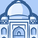# QlikView App Dev

Discussion Board for collaboration related to QlikView App Development.

Announcements
Support Case Portal has moved to Qlik Community! Read the FAQs to start exploring Support resources.
cancel
Showing results for
Did you mean:Contributor II

## SUM Distinct, Avoid Nulls with Criteria for DataSubset

Hello,

Surprised Qlik formulas do not handle zero and null very well (or as I wish).
One situation where I miss Excel.

Suppress Null checkbox is not working for me.

My current formula:

Sum(DISTINCT{\$<[Metric Name]={'Proprietary Metric'},[Latest Month]={'Y'}>}[Metric_Value])

Data and chart in attached image.

2019 and 2020 have genuine zero values (Orange Box).
2016, 2017, 2021-3 should be null on chart, but instead are displayed as zero.

Searched for formulas, but not finding an exact of If IsNull that also has specifications for a subset of data where I am specifying two criteria (in my case a particular metric and month).

Any recommendations?

Thank you kindly.

Labels (4)

• ### sum

1 Solution

Accepted SolutionsMVP

``````If(Avg({\$<[Metric Name] = {'Proprietary Metric'}, [Latest Month] = {'Y'}>} [Metric_Value]),

Sum(DISTINCT {\$<[Metric Name] = {'Proprietary Metric'}, [Latest Month] = {'Y'}>} [Metric_Value])

)``````

8 RepliesMVP

Can you try this

``Sum(DISTINCT {\$<[Metric Name] = {'Proprietary Metric'}, [Latest Month] = {'Y'}, [Metric_Value] *= {"*"}>} [Metric_Value])``Contributor II
Author

Still not functioning properly.
In need of another angle of attack.

Tried changing Null Values to read Null using SET NullValue = 'Null'; NULLASVALUE Metric_Value;
Qlik is still showing null as zero.MVP

``````If(Avg({\$<[Metric Name] = {'Proprietary Metric'}, [Latest Month] = {'Y'}>} [Metric_Value]),

Sum(DISTINCT {\$<[Metric Name] = {'Proprietary Metric'}, [Latest Month] = {'Y'}>} [Metric_Value])

)``````MVP & Luminary

I'm not sure that Qlik behaved here really differently from Excel:

Update: screenshot is attached

What do you observed hasn't much to do with the loaded data (null, empty, missing) else with the fact that you create with your chart a new table - and in there context some values could become NULL. Within a tablebox they would be displayed as NULL respecitively the '-' as optical replace of it. But if you used an aggregation like sum() the result will be 0.

If you want to avoid it you will probably need to query for the result and then changing it, maybe with something like:

if(sum(value) = 0, null(), sum(value))

- MarcusContributor II
Author

Tried suggested formulas.
Either nulls not showing, but also zero is not showing as well.

Non-ideal work around.
I converted zero to 0.001 in dataset and changed number structure to Integer.

Has to be a better solution.

Wish I could send you a gift card for this "teaching moment".

Attached Qlik File.MVP

What is a difference between Attempt 2 and Attempt 3? They look identical except the formatting for the symbol locationContributor II
Author